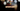# Leetcode | Solution of How Many Numbers Are Smaller Than the Current Number in JavaScript

March 29th, 2020
|

In this post, we will solve the problem how many numbers are smaller than the current number from leetcode and compute it's time and space complexities. Let's begin.

# Problem Statement

The question can be found at leetcode How Many Numbers Are Smaller Than the Current Number problem.

The problem states that we are given an array of numbers. We need to count how many numbers are smaller than the number at any given index in the array

Example -

``````Input: nums = [8,1,2,2,3]
Output: [4,0,1,1,3]``````

The first element is `8`, and elements smaller than `8` are `1,2,2,3` i.e. `4` elements are smaller than `8`. The next number is `1`, numbers smaller than `1` are none, so `0` is the output and so on.

# Solution

Let's step back and think about the problem for a minute. If the array was sorted, the index of the element would be the answer in case there are no duplicates and the first index of the element in case there were duplicates.

In the same example taken above

``````Input: nums = [8,1,2,2,3]
Output: [4,0,1,1,3]

Sorted array = [1,2,2,3,8]``````

The index of `1` is `0`, the index of `8` is `4` and so on. So all we need to do is sort the array and find the first index of all the elements.

One thing to note is, we also need to preserve the initial indices of the elements in the array because the output format has to be in the same order. So, we need to store the sorted array somewhere else.

We have discussed the approach, I urge you to go ahead on leetcode and give it another try.If you are here, it means something went wrong in implementation or you are just too lazy. In any case, let's see a simple implementation of the above logic.
``````var smallerNumbersThanCurrent = function (nums) {
const sorted = [...nums].sort((a, b) => a - b);

return nums.map(num => sorted.indexOf(num));
};``````

Let's look at the solution.

On line 2, we use the spread operator to create a new array and sort it in ascending order and place it in a variable. We need to use spread here because sort method changes the array in place and if we do it on `nums`, we will lose our initial array.

Once we have sorted array and initial array as `sorted` and `nums`, we loop over (or map over) `nums` array and get the first index of the element in the sorted array which we wanted all along. We return the array we generated from the map and that is our answer.

On submission, here are the stats

``````Status: Accepted
Runtime: 76ms
Memory: 37MB``````

## Time and space complexity

### Time complexity

We are sorting the array once, so the worst time complexity becomes O(n2).

### Space complexity

We are using extra space to store our sorted array, thus, space complexity would be O(n).

# Summary

So, we solved the how many numbers are smaller than the current number using a temporary sorted array and some array methods and finally, calculated the time and space complexities.

I hope you enjoyed solving this question. This is it for this one, complete source code for this post can be found on my Github Repo. Will see you in the next one.